KMD3
 Name:    KMD3

Multiple Choice
Identify the choice that best completes the statement or answers the question.

1.

Which does not belong?
 a.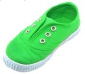c.b.d.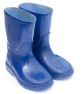2.

Which belongs with this group?a.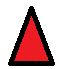c.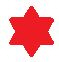b.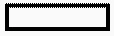d.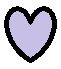3.

Which belongs with this group?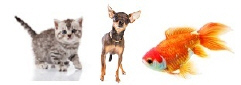a.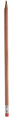c.b.d.4.

Which does not belong?
 a. T c. S b.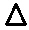d. A

5.

Which does not belong?
 a. H c. 5 b. 7 d. 2

6.

Which belongs with this group?a.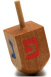c.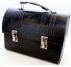b.d.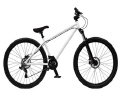7.

Which belongs with this group?a.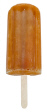c.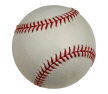b.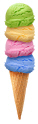d.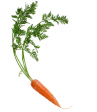8.

Which does not belong?
 a.c.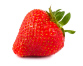b.d.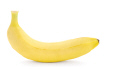9.

Which belongs with this group?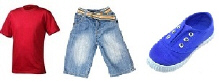a.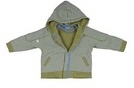c.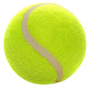b.d.